Synergetics. Etudes 70.

Is Dedicated to Centennial of Birthday of Outstanding Scientist, Professor

Basin Abram M.

Basina G. I., Basin M. A.

SIC ”Synergetics” of Saint-Petersburg Association of Scientist and Scholars.

Etude 5.

Algebraic and Spiral Complex Numbers.

April 24. 2010.

Here we shall introduce one of possible modifications of complex numbers, using of which allows, if it is necessary, to consider power functions of complex variable with complex degrees of power as functions, having a single meaning, and consequently to apply to there investigation all apparatus of contemporary analysis.

Every complex number has as minimum two possible representations: algebraic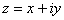and exponential -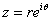. To each algebraic representation of complex number corresponds enumerable set of exponential representations, in which value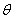differs on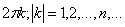. Shall we suppose, following B. Riemann and G. Weil, that there is any screw spiral structure, crossing complex plane on positive axis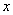with aspiring to zero step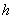[3-5]. To every point of such spiral we shall correspond spiral complex number, which may be described with the formula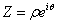. Value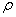characterizes distance from the point of spiral surface to the axis, perpendicular to the planeand passing through the point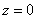. At such geometric interpretation all points, corresponding to the spiral complex  numbers, having meanings of, differing onlay on the one straight line, which projection on plane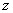is one algebraic complex number, where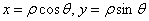. To every spiral complex number corresponds one algebraic complex number. But to every algebraic complex number correspond enumerable set of spiral complex numbers. Shall we introduce in consideration another complex plane, which corresponds to the field of algebraic complex numbers, which will get, if we shall formal take the operation of logarithm from every spiral number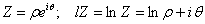. Function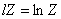and inverse to it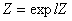are one-for-one functions, reflecting each on other area of determination of spiral numbersand complex plane. Each horizontal streak of the areawith height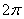corresponds to one blade of spiral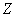.

Shall we consider in the areatotality of one-for-one complex linear reflections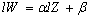. As coefficients of such reflections we shall take the fields of algebraic complex numbers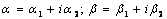. These reflections are the one-for one reflections in all points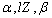, except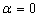.

Shall we introduce another one-for-one reflection of complex plane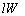on the area of spiral complex numbers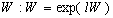. Then we shall receive one for one reflection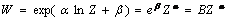/ Constant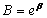also lays in the area of determination of spiral numbers. So the multi-ciphered power function with complex numbers of degree at the consideration of it in the area of determination of spiral numbers is one-for-one reflection. So we can to do with it all operations how with power functions, determining in the area of real varieties. Introduction of spiral complex numbers creates the conditions for the development of power geometry with algebraic complex power numbers. In any cases, when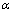is integer we may work with projections of spiral numbers on complex planes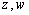.

Being in frames of algebraic complex numbers we have a risk to lose possible elements of volume of points, on which reflects any power function given algebraic complex number. Arise multi-ciphered and infinity-ciphered functions and with them concept of variety of choice of any branch of multi-ciphered reflection.

It is the problem of relation of built mathematically construction with real objects, which are describing with this mathematically construction.  We not always “see” the spiral complex number, often we observe its projection on the complex plane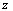and describing with  usual power functions dynamics of system “seems” us as bifurcation dynamics.

While built by us one-for-one power functions are the exponents of the algebra of linear reflections on the complex numbers, then in the area of determination of spiral numbers naturally may be introduced multiplication of spiral complex numbers as the spiral analog  of summarizing  of powers of exponents of corresponding algebraic complex numbers. Analogous statement may be done about multiplication of power functions of any spiral complex variable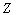. Shall we have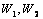-two power functions of spiral variable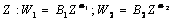.

Then function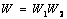also will be one-for-one power function from.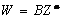, где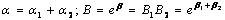.

With analogous matter we can prove, that exponentiation of power function in complex degree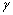generates new degree function with power, equal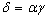, and coefficient representing spiral number, which is equal to the coefficient of primary spiral number exponented in the degree.

It is more difficult to introduce the summarizing  of spiral complex numbers, and the summarizing of power monomers. This problem may be called the problem of Lagrange  . We shall show only the ways of decision of this problem. Shall we have two spiral numbers or two power functions of spiral variable. We must to determine the sum of this spiral numbers or functions.

In the area of spiral numbers to give determination of the sum if difficult. But we may to project spiral numbers on the plane of algebraic complex numbers and to compute the sum of two algebraic complex numbers. If we shall consider that sum of algebraic complex numbers is the projection of spiral complex number, which is the sum of spiral complex numbers, then our determinasion allows with the accuracy of infinity values of meanings of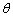, districting one for one on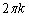, such sum to determine. More detail this problem will be considered in th next Etude.

References.

1.Riemann B. Theorie der Abelschen Funktionen. Borhardt’s Journ. für reine und angewandte Math. 54.). 1857. Werke. Leipzig 1876.S.81-135.

2. Weyl H. Die Idee der Riemanischen Fläche. Leipzig-Berlin. 1913 (1-ste Aufl.). 1923 (2-te Aufl.). Stuttgart.1953 (3-te Aufl.)

3.Шабат Б. В.Введение в комплексный анализ. Ч.1. Функции одного переменного. М. 1976

4.Стоилов С. Теория функций комплексного переменного. В 2 томах. Основные понятия и принципы. М.:1962.

5. Басин М. А. Спиральные числа. Степенные особенности. Волны. Вихри. Грибовидные структуры. Транспортно-информационные системы. Международная междисциплинарная научно-практическая конференция: «Современные проблемы науки и образования». Керчь 27.06-4.07.2001. Ч.1 Харьков. 2001. С.12-13.

6.Арнольд В.И., Авец А. Эргодические проблемы классической механики. Ижевск 1999